﻿ Evaluating the Performance of Biometric Identification Systems Using the Beta-binomial Distribution ModelPublications are Open
Access in this journal
Article Versions
Export Article
• Normal Style
• MLA Style
• APA Style
• Chicago Style
Review Article
Open Access Peer-reviewed

### Evaluating the Performance of Biometric Identification Systems Using the Beta-binomial Distribution Model

Arnold Kiura Njuki , Thomas Mageto, Anthony Ngunyi
American Journal of Applied Mathematics and Statistics. 2022, 10(1), 14-21. DOI: 10.12691/ajams-10-1-3
Received February 05, 2022; Revised March 07, 2022; Accepted March 15, 2022

### Abstract

Biometric authentication system has become a mainstream solution across industries and devices. From securing highly confidential data to unlocking smartphones, biometrics have eliminated the hassle of remembering multiple complex passwords and PINs. It means that nobody can gain access to a device or system without your presence. This paper discusses a method which could be used in the testing process of biometric systems on the side of users and customers. Large –scale biometric systems traditionally undergo a series of tests beyond technology and scenario testing. These large-scale system tests are typically at the system level, not just the biometric subsystem level, and occur multiple times in the life of a system in such forms as factory acceptance tests before shipment, site or system acceptance tests before initiating operations, and in- use tests to ensure that performance remains at acceptable levels and/or to reset thresholds or other technical parameters. The conventional statistical methods use the binomial distribution to estimate the expected number of failure, but in the field of the biometrics the probability parameter can’t be constant which means that it is necessary to describe a process. The results have shown that the probability is characterized with two parameters of the beta distribution, and these are predictable from a smaller sample of the investigated population with the maximum likelihood method.

### 1. Introduction

Biometric system has become the benchmark technology for its potential to reduce the limitations of existing security systems and eliminate data breach activities. Organizations and app companies undergo numerous tests before and after deployment. Prospective biometric technologies are examined for underlying strengths of their technology/modality, usability, and accuracy. This testing is performed under optimal, controlled conditions for all relevant parameters that can affect performance.

Since organizations have had existing biometric technology in place and a substantial amount of experience with biometric industry, their mindset is that a threshold has been set for performance in both error rates and throughput. Parameters like technology construction and architecture, component mean time between failures and theoretical throughput are extrapolated based on the results of tests.

Before the era of the biometrical identification the main question during the authentication process was that: the given ‘key’, (RFID chip, PIN code, etc.) “Is able to open the lock”? This ‘key’ was mostly constructed by a binary code. This code, excluding that how it is encrypted, gives only one suitable solution which can be totally selective. In contrast, biometric authentication methods serve as a form of two-factor authentication (2FA) or multi-factor authentication (MFA), either by combining multiple biometric patterns or in conjunction with a traditional password or secondary device that supplements the biometric verification. The user’s biometric data is matched against all the records in the database as the user can be anywhere in the database or he/she actually does not have to be there at all. The specific question addressed in this section is “How likely is the verification system to make an incorrect decision?” The phrasing of the question implies a likelihood associated with errors. In other words, errors do not occur deterministically, but rather in a probabilistic manner.

There is the need for the testing of biometric identification devices that utilize more than one individual and, for efficiency, that individual is tested more than once. The goal of test is to assess how the Biometric device would perform when implemented on a population of users. Binomial model is not appropriate when the probability of “success” varies from individual to individual. Probability of success, p, the usual binomial parameter is not the same for each user. Thus, the binomial is not appropriate for assessing the performance of a Biometric identification device when combining outcomes from multiple users. The Beta-binomial model or, more formally, the product Beta-binomial allows for variability in the probability of success among individuals and that allows for the possibility that trials by a given individual are not independent. The probability of the possible events is not constant, but it follows a special distribution. This distribution of the probability parameter (p) is determined by two parameters –α, β.

### 2. Literature Review

For any biometrics identification device, assessing its matching performance is often critical to the success of the product from the viewpoint of both the vendor and the consumer. There are lots of biometric techniques available nowadays. A few of them are in the stage of the research only (e.g. the odor analysis), but a significant number of technologies is already mature and commercially available, examples include: fingerprint, finger geometry, hand geometry, palm print, iris pattern, retina pattern, facial recognition, voice comparison, signature dynamics and typing rhythm (U.K. Biometrics Working Group, 2000)) 9.

The goal of a biometric device is to accurately determine whether or not you are who you say you are. There are several factors that go into a ‘good’ biometric device. Hong, Wan, Jain, 14 suggest that a biometric should possess the following characteristics: universality, uniqueness, permanence, collectability, performance, acceptability, and circumvention. Universality means that as many people as possible should have the biometric in question. Not every person has a right index finger, so that a biometric device based solely on this will not be universal. Next, uniqueness implies that each person should have a different version of the biometric. Fingerprints are generally thought to be unique. Permanence is the condition that the biometric should not change over time. A biometric device based on facial recognition is not ideal in this sense because people change their hair, they grow beards and they get wrinkles. The ease with which a biometric can be captured is its collectability. It might be possible to create a biometric device based upon your EEG, but it would be difficult to capture that information quickly and easily. On the other hand, a fingerprint or an iris is fairly exposed and, therefore, easily collectible. Performance measures how easy a particular biometric is to use and implement. Acceptability is the degree to which there is public acceptance of the biometric for identification purposes. Fingerprints are a prime example of a biometric with high acceptability, since they have been used for centuries as a method of identification. Finally, circumvention is the amount of work need to fool the system. Signatures are notoriously easy to reproduce, whereas creating a copy of a fingerprint is far more difficult. Discussing some of the commonly used Biometric Identification Devices (BID):

2.1. Finger Prints

Fingerprint identification is perhaps the oldest of all the biometric techniques. Their use in law enforcement since the last century is well the oldest known. Optical fingerprint readers are the most common at present. They are based on reflection changes at the spots where the finger papilla lines touch the read surface. Optical fingerprint readers cannot be fooled by a simple picture of a fingerprint, but any 3D fingerprint model makes a significant problem, all the reader checks is the pressure. A very good example of fingerprint reader is the IEVO-M microTM Biometric Fingerprint Reader which is a compact fingerprint reader designed for internal use only, looking to secure small to medium sized facilities. It uses an optical sensor and delivers a fast and reliable biometric solution saving time and costs to any business. The fingerprint biometric authentication methodrely on partial information to authenticate a identity. For example, a mobile biometric device will scan an entire fingerprint during the enrollment phase, and convert it into data. However, future biometric authentication of the fingerprint will only use parts of the prints to verify identity so faster and quicker. The fingerprint reader is enabled for 128-bit data transmission and provides highly accurate and quality images to be transferred to the Control Board, where the data is securely stored. Here it performs 1: N, matching up to 50,000 (10,000 standard) fingerprint templates.

2.2. Facial Recognition

Facial recognition is the most natural means of biometric identification. The method of distinguishing one individual from another is an ability of virtually every human. Any camera (with a sufficient resolution) can be used to obtain the image of the face. Any scanned picture can be used as well. The image processing and facial similarity decision process is done by the computer software. The accuracy of the face recognition systems improves with time, the current software may often find “a face” at an incorrect place. This significantly makes the results worse. Better results can be achieved if the operator is able to tell the system exactly where the eyes are positioned. The systems also have problems to distinguish very similar persons like twins and any significant change in hair or beard style requires re-enrollment. The quoted accuracy of facial recognition systems varies significantly, many systems quote the crossover accuracy of less than one percent.

2.3. Iris Scan

Research shows that the matching accuracy of iris identification is greater than of the DNA testing. The iris scanner does not need any special lighting conditions or any special kind of light (unlike the infrared light needed for the retina scanning). The iris scanning technology is not intrusive and thus is deemed acceptable by most users. The iris pattern remains stable over a person’s life, being only affected by several diseases. Once the gray-scale image of the eye is obtained then a software tries to locate the iris within the image. In the decision process the matching software given 2 iris codes computes the Hamming distance based on the number of different bits. The Hamming distance is a score (Within the range 0 – 1, where 0 means the same iris codes), which is then compared with the security threshold to make the final decision. Computing the Hamming distance of two iris codes is very fast (it is in fact only counting the number of bits in the exclusive OR of the two iris codes). Modern computers are able to compare over 4 000 000 iris codes in one second. An iris scan produces a high data volume which implies a high discrimination (identification) rate. The iris recognition is the fastest identification out of all the biometric systems I could work with. It is hard to encounter a false acceptance (the database was not very large, however) and the false rejection rate is reasonably low. The manufacturer quotes the equal error rate of 0.00008%, but so low false rejection rate is not achievable with normal (nonprofessional) users.

2.4. Hand Geometry

Hand geometry is based on the fact that nearly every person’s hand is shaped differently and that the shape of a person’s hand does not change after certain age. Hand geometry systems produce estimates of certain measurements of the hand such as the length and the width of fingers. Various methods are used to measure the hand. These methods are most commonly based either on mechanical or optical principle. The latter ones are much more common today. Optical hand geometry scanners capture the image of the hand and using the image edge detection algorithm compute the hand’s characteristics. Hand geometry scanners are easy to use. Hand geometry does not produce a large data set (as compared to other biometric systems). Therefore, given a large number of records, hand geometry may not be able to distinguish sufficiently one individual from another. The size of the hand template is often as small as 9 bytes. Such systems are not suitable for identification at all. The verification results show that hand geometry systems are suitable for lower level security application. The manufacturers advertise the crossover accuracy about 0.1%. These numbers are difficult to obtain in reality. FAR of 3% and FRR of 10% at the middle security threshold are more realistic. The verification takes about one second. The speed is not a crucial point because the hand geometry systems can be used for verification only.

### 3. Methodology

We have discussed some of the common biometric technologies, at the present there is no consensus on a methodology for assessing the performance of a biometric device when two or more individuals are tested. The binomial distribution is incorrect when more than one individual attempts to match. The matching performance is usually measured in terms of false accept and false reject rates. I will refer to users that are enrolled in the database as genuine users and I will refer to users who are not enrolled in the database as imposters. Thus, the matching performance describes how well the system allows access to genuine users and denies access to imposters.

When an individual presents their biometric, the ‘image’ is processed and matched against one or more stored templates from the database. The number of comparisons depends upon the mode that the device uses. There are two basic modes of operation. The first is verification or one-to- one mode. In this mode, some identifier such as a name or an ID number is given to the system and it verifies that your biometric matches the biometrics stored under your name. The second mode of operation is identification or one-to-many mode. Under this scenario, the biometric system compares the presented biometric to the entire database looking for a match. Though these systems have very different methodologies, their performance is measured in the same way.

To make this discussion more precise, consider the population of match scores all attempts by genuine users and let fgen(x) represent the density of this distribution. Similarly, consider the population of match scores for all attempts by imposters and let himp(y) be the density for this distribution. Then the false rejection rate (FRR) is the probability that T is greater than λ given that T comes from the distribution of genuine user scores. The false acceptance rate (FAR) is the probability that T is less than λ given that the score T comes from the distribution of imposter’s scores. 10 Symbolically,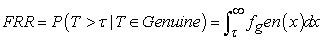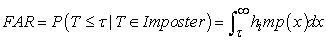The threshold, λ, can be set so that we have some control over the values that the FAR and FRR will take. However, note that as λ increases that the FRR will decrease and the FAR will increase. Likewise, as λ decreases the FRR will increase and the FAR will decrease. In a practical setting, we are often interested in estimating the FAR and FRR for a particular biometric device. Given λ and a sample from both genuine users and imposters we can create estimates for the FRR and FAR, in the following way: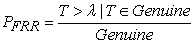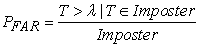where PF RR where is an estimator of the FRR, PF AR is an estimator of the FAR, Genuine is the total number of genuine user scores and Imposter is the total number of imposter scores. The security thresholds of biometric systems is measured depending on variability of FAR and FRR. Once biometric data is matched against all records we hypothesize that there could be sufficient overlap between various biometrics to allow a hacker or imposter to access a device at a certain percentage of time. The variability is the security threshold or security level. When the variability is small then the security threhold is high. This means f you try to reduce the FAR to the lowest possible level, the FRR is likely to rise sharply. In other words, the more secure your access control, the less convenient it will be, as users are falsely rejected by the system. The same also applies the other way round. When the variability allowed is great then the security level is low. Do you want to increase user convenience by reducing the FRR? In this case the system is likely to be less secure (higher FAR).

BIDs have been investigated by statistical methods for easier reproducibility and usage. The results have shown that the less the number of the investigated variables the higher the willingness to apply the method by the side of customers. I had to involve an automatic technique which is easily adoptable in the practice. The theory of the process is the following:

In the first step it is necessary to pick a representative sample of the multiplicity. In this case that means a small group of those individuals who are using the investigated access point. The access point used was the fingerprint reader despite several BIDs in the market. The human identification patterns (HIP) are not permanent, those are continuously changing in time, sometimes day by day or person by person. To determine the exact value of failure rates the examination of these devices has to be regular and systematic. Biometric testers are very interested in knowing how large of a sample they need to take. We determine two sample sizes: M, the number of individuals to be sampled and, ni, the number of times that each individual should be tested. . It is proven there aren’t two totally equal samples in the biometrics, even if they have been recorded from the same person in the same time.

In the field of biometrics we have to count with continuously changing user attribution and environment, thus the numbers of the failures (not recognized HIP) are variables. But this variety is not chaotic, it is possible to be described by the beta-binomial distribution.

In the conducted experiments we examined how big the possibility of the failures. Six tests have been repeated six times, and each time we noticed the number of the failed identification. These rounds were subscribing the distribution of the possible failures. However I had to find the method which is able numerically characterize this distribution. As it was mentioned above this distribution is the beta-binomial distribution, so the exact task in the second step was that to find the right way to determine the parameters in each of the beta distributions at every subject. These results individually are not able to classify the goodness of the BID (fingerprint reader), just showing whether the methodology works or not.

We made differences between the failures by the level of the individuals and tests. With the parameters of the Beta – Binomial distribution it is possible to calculate the beta-binomial density function that shows the possibility the different cases of the failures. The individual density function compared with the density function of the aggregated data, it is possible to establish whether there is a subject with very poor pattern or the device’s gone wrong. According to the beta-binomial parameters it is possible to estimate the probability of each failure cases(e.g. one, two, or more failure in a ten size sample), and even possible to give the expected value and dispersion of the distribution. The convectional FRR is calculated as follows: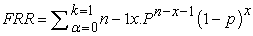where k is the minimum number of minutiae to access and p is probability of failure. In contrast with the FRR the expected values and the variance of the beta-binomial distribution is the following: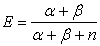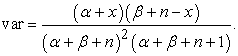After while there has been observed n different events, the number of the adverse events is x, and so the number of the not false identifications is n-x, the corrected equation is the following: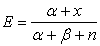A simple failure can be tolerated in the intensive daily use, but if the FRR significantly increases, then the effectiveness of the device brakes down, which could lead in a marginal case to turning off. The Beta-binomial distribution that is described in this paper is a generalization of the binomial distribution that allows for correlation between trials for a given individual. Consequently, the Beta-binomial can be an appropriate model where the binomial is inappropriate.

3.1. Mathematical Background of Beta-Binomial Distribution

The Beta-binomial is derived in the following manner 6. Suppose that we have m individuals and each of those individuals is tested ni times, where i = 1, . . . , m. Assume that,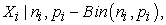where Xi is the number of successes, and that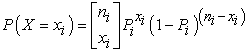We then further model each of the pi as conditionally independent draws from a Beta distribution. The Beta distribution is a continuous distribution on the interval [0,1] and it is parameterized with two quantities, α and β. Letting pi have a Beta distribution, the probability density function is then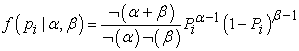The mean and the variance for a Beta random variable are given by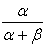and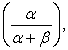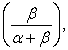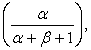respectively. If we let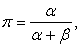then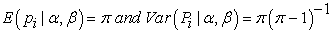The joint distribution is then,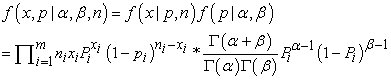(3)

where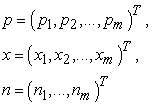Now inference for this hierarchical model should be focused on α and β, since they define the overall probability of success. Consequently, we can integrate out the pi’s because they are now nuisance parameters. Thus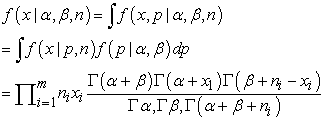(4)

Equation (4) is referred to as a joint Beta-binomial distribution or product Beta-binomial distribution. Let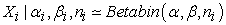represent Xi coming from a Beta-binomial distribution conditional on the parameters α, β and ni. Thus, we assume that the Xi’s are conditionally independent draws from a Beta-binomial distribution with parameters α, β and ni. Under that distribution,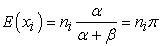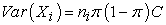where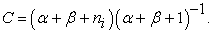For the determination of the Beta-Binomial parameters, α and β we used the maximum-likelihood method. The basic underlying idea for ML estimation is to find the parameter value most likely to have produced the observed data. For example, given data Y from a sampling distribution f (Y|θ) with parameter θ, the likelihood function L(θ|Y) is the sampling distribution treating the data as known and the parameter as unknown. Note that both Y and θ are potentially vector- valued.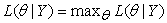We also have estimators based on Mean and zero (mean zeros) which has high efficiency when fitting BB to reverse j-shaped distributions, also estimators based on first two sample moments (2 moments) and estimators based on mean and ratio of ones to zeros (1 moment-1 probability)

3.2. Estimators Based on Mean and Zero (mean-zeros)

Chatfield, Goodhart 13 conjectured that the method of mean and zeros would have high efficiency when fitting the BB to reverse J-shaped distributions. Let p0 denote the sample proportion of observed zeros and µ the sample mean. Then the estimators of β and α based on po and µ are obtained by solving the equations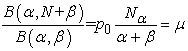(1.1)
3.3. Estimators Based on First Two Sample Moments (2-moments)

Let μj denote the j-th sample factorial moment and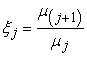setting j = 0, 1 in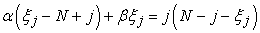and solving the two equations yields the estimators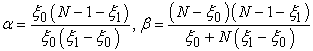(1.2)
3.4. Estimators Based on the Mean and the Ratio of Ones to Zeros (1 Moment-1 Probability)

Since the estimators in eq (1.1) involve a nonlinear equation for the zeros, it is tempting to replace it by a linear equation involving the ratio of ones to zeros obtained from equation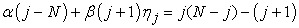with j = 0. Let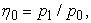the ratio of the proportion of observed ones to the proportion of observed zeros. Then a simple estimator can be obtained by solving the two linear equations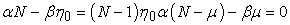where µ is the sample mean. This yields the estimators.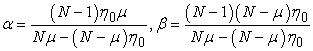(1.3)

We can use the R-package BBest (y,m,method=”MLE”) where

• y-reponse variable which follows Beta-Binomial distribution.

• m-maximum score number in each beta binomial observation.

• method-the method used for performing the estimation of the probability and dispersion parameters of a Beta-Binomial distribution. ”MM” represents method of moments-Default and ”MLE” is Maximum Likelihood Estimation.

### 4. Data Analysis Discussion

The practical probability of malfunctions can be estimated for each of the users, and it is also able to be estimated for a whole and complex system. In this paper the chosen individual level was, due to comparing the convenience statistical analyzing with the more practical examinations. For this research an iEVO micro fingerprint reader used in our Huduma center (Kirinyaga County) has been chosen. I assume that m individuals were tested for each of k times. For simplicity, suppose that each individual was tested the same number of times, so that ni = k for each individual, i.

We have eight subjects (volunteer) have been used in the tests, but finally only four of them’s results were able to used in the algorithm. The rest had trouble with the appropriate usage of the fingerprint reader.

In our tests we got the results above. Each test was repeated ten times per each user, so the Table 2 shows how many times 0, 1, 2 or more failures occurred in each test which contains six tries.

As shown, the rate of the failures during the recognition (FRR) is about 18% (71 out of 400). This is significantly higher than the manufacturer (0.1%). The manufacturer gave the original (empirical) data for characterize the goodness of the device; FRR < 0.1% and FAR < 0.00001%.

The beta parameters were calculated for each user and we presented the calculated beta distribution density functions as follows.

• Figure 1. Beta Distribution density Function for subject 1
• Figure 2. Beta Distribution density Function for subject II
• Figure 3. Beta Distribution density Function for subject III
• Figure 4. Beta Distribution density Function for subject IV

The beta density functions for the summarized data and the average value is added as well in Figure 5 and Figure 6.

• Figure 5. Beta Distribution density Function for the Average value
• Figure 6. Beta Distribution density Function for the Summed values’ parameters

All the test when the number of the failure is zero or quite small is significantly higher than the more times failed. The usual statistic uses the binomial distribution to estimate the average number of failures. To compare the conventional method with this examined method we used the average number of the experienced failures as parameter p in the binomial equation. In the betabinomial method the probability of zero failures is about 35%, in contrast with the normal binomial method, where it is 10-15%. Although the tests were done on a smaller population, the necessary minimal failed events have been detected on 99% level of confidence, according to the Doddington formula cited in (Doddington, Liggett, Martin, Przybocki, Reynolds, 1998) 11. In the testing of smaller populations usually the deformity means that the experienced failure number is smaller than the statistically expected. The Doddington formula helps to correcting this deformity. So the problem that origins from the small population slightly corrected, thus we could focus to compare the significant difference between the normal binomial and beta-binomial distribution. Final probability values in the different methods (average of the subjects and the accumulated cases) are shown in the Figure 7 and Figure 8 below.

• Figure 7. Probability values for the Average of the subjects
• Figure 8. Probability values for the Summed values’ of the subjects

### 5. Conclusions

The Beta-binomial distribution accounts for the extraneous variability in this scenario. Several authors including I have noted that a binomial distribution is not appropriate for biometric data under most circumstances. The problem with using the binomial distribution to describe the data is that it does not allow for intraindividual correlation in testing. [This is the correlation in repeated measurements for the same subject]. The Beta binomial distribution is appropriate for describing biometric data because it has the flexibility to model the correlation of observations by the same individual. The beta binomial distribution draws conclusions on false rejections and false acceptances. An ideal system has no false rejections and false acceptances whereas the real system those numbers are non-zero and depend on security level or security threshold. The false rejection rate decreases as the variability increases. The failed rejection depends on more variables, for which there doesn’t exist an explicit formula. Because the failures come from different set of mistakes and statistical uncertainty. We can conclude from the information above that the Huduma center will often prioritise user convenience over security. This way may be acceptable to users. The thinking behind this: We don’t want people to have to queue up at the door because the system is not working properly.

### References

  Rand R Wilcox, “Estimating the Parameters of the Beta- Binomial Distribution,” University of Southern California. (2016). In article  Gabor Á. Werner, László Hanka Ph.D, “Using the Beta-Binomial Distribution for the Analysis of Biometric Identification,” Óbuda University. (2015). In article View Article  PubMed  Dan Navarro, Amy Perfors, “An introduction to the Beta-Binomial model,” COMPSCI 3016: Computational Cognitive Science, University of Adelaide (2014). In article  László Hanka, “Mathematical Methods in Biometrics,” University of Óbuda, (2012). In article  In.van Tilborg H.C.A., Jajodia S. “Biometric Testing. In: (eds) Encyclopedia of Cryptography and Security.” Springer, Boston, MA. (2011). In article View Article  J.Tong and D.Lord. “Beta-Binomial Models-CMRSC”. (2007). In article  Gammasi M, Lazzaroni M,Mishori M, Piuri V, Sana D, Scotti F. “Accuracy and performance of Biometric Systems”. (2004). In article  Dass SC, Zhu Y, Jain AK,Anal Mach Intell, “Validating a biometric authentication system: sample size requirements”. IEEE Trans Pattern (2006). In article View Article  PubMed  U.K. Biometrics Working Group. “Best practices in testing and reporting performance of biometric devices”, available in www.cesg.gov.uk/biometrics, (2000). In article  Michael E. Schuckers. “Using the Beta-binomial distribution to assess the performance of a biometic device” Submitted to Pattern Recognition (2003)[Online]. In article View Article  G. R. Doddington, W. Liggett, A. F. Martin, M. Przybocki, and D. A. Reynolds, “Sheep, Goats, Lambs and Wolves: A statistical analysis of speaker performance in the NIST speaker recognition evaluation”. (1998). In article  S. Silvey. “Statistical Inference” Halsted Press, New York. (1975). In article  Chatfield and Goodhart. “Applied Statistics” 19, 240-250 (1970). In article View Article  Lin Hong, Yifei Wan, and Anil Jain. “Fingerprint Image Enhancement: Algorithm and Performance Evaluation” Pattern Recognition and Image Processing Laboratory Department of Computer Science, Michigan State University. 4-6 (1998). In article

Published with license by Science and Education Publishing, Copyright © 2022 Arnold Kiura Njuki, Thomas Mageto and Anthony NgunyiThis work is licensed under a Creative Commons Attribution 4.0 International License. To view a copy of this license, visit http://creativecommons.org/licenses/by/4.0/